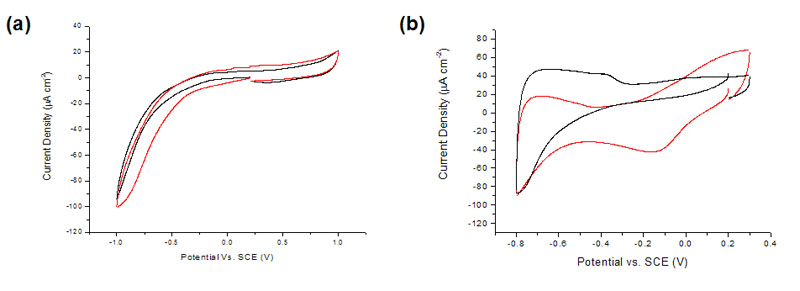# Dissolved Oxygen Detection

Pt Nanoparticle-pBDD Composite Electrodes

Oxygen terminated pBDD macrodisk electrodes (1 mm diameter) were shown to be electrochemically insensitive to dissolved oxygen (Figure 1a).  In comparison, a large oxygen reduction peak can be observed at -0.175 V using a Pt electrode (Figure 1b).To improve the sensitivity of pBDD toward amperometric oxygen detection, decoration of the surface with electrodeposited Pt particles was explored.  The main aim was to achieve minimal background current from processes at the Pt particles, which scale with surface area, while obtaining a well-defined and quantitative signal for oxygen reduction.  CVs of oxygen reduction in aerated solution for pBDD electrodes subjected to Pt electrodeposition at -1.0 V for times in the range 0.1-60 s were carried out (Figure 1c).  Electrodeposition potentials more negative than -1.0 V gave noisy chronoamperometric signals due to concomitant H2 evolution at the deposited Pt.  Optimum deposition parameters of -1.0 V for 5 s were chosen for subsequent studies as these conditions gave low background currents, due to the reduced amount of Pt on the surface, with good oxygen amperometric detection sensitivity.(C)Figure 1. Cyclic voltammograms for the reduction of dissolved oxygen at a (a) 1 mm diameter pBDD disk electrode (b) 3 mm Pt disk electrode, in nitrogen saturated (black) and areated (red) 0.1 M KNO3 at 100 mVs-1 and (c) for different Pt deposition times.

Surface Characterisation

FE-SEM and AFM were used to characterise the composite electrode surface for the optimised deposition parameters.  With the In-Lens detector on the SEM it is possible to resolve the underlying pBDD grain structure as well as the Pt particles. SEM images of the composite electrodes show Pt NPs depositied randomly over the pBDD surface with no evidence of preferential deposition at grain boundaries (Figure 2).  A difference in the density of particles between grains of varying conductivity can also be observed.Figure 2. FE-SEM images of a Pt NP-modified pBDD electrode, for Pt deposition parameters of -1.0 V for 5 s at two magnifications.

The morphology of the Pt particles was further investigated using tapping-mode AFM.  These images of the electrochemically depositied Pt NPs show that for low conductivity grains the chosen deposition parameters give a particle density of ~130 Pt NPs per square um with a mean size of ~3 nm (Figure 3a).  Whereas higher conductivity grains have a particle density of ~340 Pt NPs per square um with a mean size of ~1 nm (Figure 3b). The SEM and AFM data are consistent with our previous studies of electroeposited metals on pBDD, where smaller particles at high density appear on the more conducting grains.  It is important to note that the close spacing of the Pt NPs means that on the time scale of typical voltammetric measurements there will be considerable diffusional overlap between particles. Thus the electrode behaves as a conventional planar electrode.Figure 3. AFM (tapping mode) height images of a Pt NP-modified pBDD electrode, for Pt deposition parameters of -1.0 V for 5 s at two different grains with varying conductivity.

Dissolved Oxygen Sensor

The sensitivity of the composite electrode to varying dissolved oxygen concentrations, from 0 - 100 % oxygenated, for different pH conditions (pH 4, 5.5, 7.5 and 10) was investigated.  This was done by flowing varying ratios of oxygen and nitrogen gas into solution and performing cyclic voltammetry (Figure 4a).  A clear oxygen reduction peak was observed at -0.195 V, which showed a strong response to the dissolved oxygen concentration.  A plot of peak current against oxygen concentration shows a strong linear correlation (Figure 4b).Figure 4. (a) CVs for the reduction of oxygen in 0.1M KNO3 and H2SO4 (pH 4) at a Pt NP-modified pBDD electrode, at percentages of oxygen of 0 (smallest current), 10, 20, 30, 40, 50, 70, 90, and 100% (largest current). (b) Plot of background corrected peak current against dissolved oxygen concentration.

Chronoamperometry was also employed and showed that over a reasonable time scale, the current plotted against t-1/2, for all oxygen concentrations and all pH solution conditions, yielded a straight line as predicted by the Cottrell equation (Figure 5a).  Gradients from the Cottrellian analyses (background corrected), as well as the current at 3 s, versus the oxygen concentration for pH 4, 5.5, 7.5 and 10 were plotted and a linear relationship was observed (Figure 5b).  These results show that oxygen concentrations as low as 1 ppb should be detectable over a range of pH conditions.  Current work is focused on the stabilisation of the Pt NPs for precise long term detection.Figure 5. (a) Chronoamperometric current plotted against time-1/2 for 0.1 M KNO3 solution with 30% oxygen at pH 4. (b) Cottrell gradient (black) and the current taken at 3 s (blue) plotted against the dissolved oxygen concentration in 0.1 M KNO3 solution for (0) pH 4, (O) pH 5.5, (4) pH 7.5, and (3) pH 10.

## References

1. L. Hutton, M. E. Newton, P. R. Unwin and J. V. Macpherson, Anal. Chem., 2009, 81(3), 1023–1032.